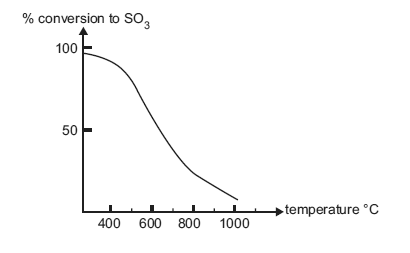Industrial chemistry (sulfuric acid) exercise (2006 VCE) One of the steps involved in the industrial preparation of sulfuric acid is the oxidation of sulfur dioxide to sulfur trioxide according to the equation 2SO2(g) + O2(g) 2SO3(g) a. Give the name or formula for the catalyst that is most widely used for this reaction. Solution The graph below shows the percentage conversion of sulfur dioxide to sulfur trioxide at equilibrium at 1 atm pressure and various temperatures. There is almost complete conversion of sulfur dioxide to sulfur trioxide at 300°C. However, this reaction is performed at a higher temperature of approximately 450°C in industry. Why?SolutionExplain why i. high pressures would increase the equilibrium yield of sulfur trioxide in this reaction. Solution ii. atmospheric pressure is usually used in industry, even though high pressures increase the equilibrium yield of sulfur trioxide in this reaction. SolutionA chemical reaction that involves sulfuric acid occurs in each of the following situations. Write a balanced chemical equation for each reaction, showing the states of all reactants and products. i. Dilute sulfuric acid is added to sodium carbonate solution Solution ii. Sulfur trioxide gas is bubbled through concentrated sulfuric acid. Solution iii. A piece of zinc metal is added to 6 M sulfuric acid. Zinc ions are formed as well as an oxide of sulfur in which sulfur exhibits an oxidation state of +4. Solution